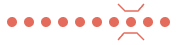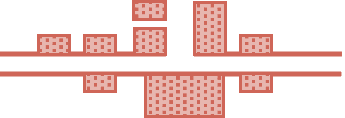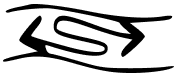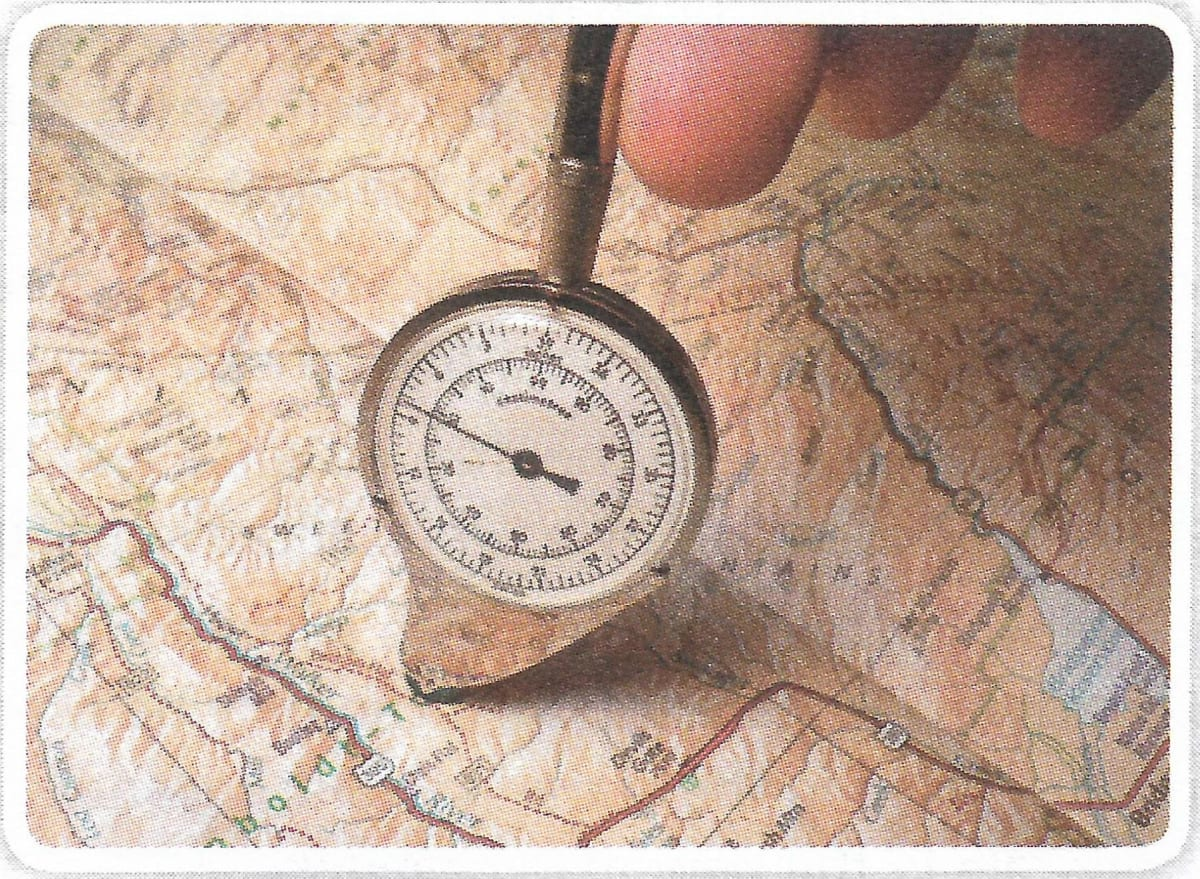# Representation of Geographical Features

## Get Set

#### Question 1

The history of map-making is given below. Number the events in the sequence in which they took place.

• Gerardus Mercator was the first to draw a world map in 1538.
• Modern map-making was started by the ancient Greeks. Ptolemy in the second century CE was the first to make a collection of maps called Geographia.
• Remote Sensing was introduced in the 1970s. It led to the development of Geographic Information System or GIS.
• Later, the first modern atlas Theatrum Orbis Terrarum was published in 1570.
• The history of maps dates back to 4,500 years. During that time maps were pictorial and were drawn without any scale or accuracy.
• During the Medieval period, the Arab geographers excelled in map-making.
• The earliest known map, that is still preserved, is in the form of a small baked clay tablet. It was made in about 2300 BCE and shows a part of Babylonia (present-day Iraq).
• The introduction of aerial photography in the nineteenth century started a new phase in landform surveys which enhanced map-making.

The correct sequence is as follows:

1. The history of maps dates back to 4,500 years. During that time maps were pictorial and were drawn without any scale or accuracy.
2. The earliest known map, that is still preserved, is in the form of a small baked clay tablet. It was made in about 2300 BCE and shows a part of Babylonia (present-day Iraq).
3. Modern map-making was started by the ancient Greeks. Ptolemy in the second century CE was the first to make a collection of maps called Geographia.
4. During the Medieval period, the Arab geographers excelled in map-making.
5. Gerardus Mercator was the first to draw a world map in 1538.
6. Later, the first modern atlas Theatrum Orbis Terrarum was published in 1570.
7. The introduction of aerial photography in the nineteenth century started a new phase in landform surveys which enhanced map-making.
8. Remote Sensing was introduced in the 1970s. It led to the development of Geographic Information System or GIS.

## Quick Quiz

#### Question 1

The network of parallels and meridians on a map is called a ............... .

The network of parallels and meridians on a map is called a grid.

#### Question 2

On a topo sheet green is used to show cultivated areas. True or False?

False

Corrected Statement— On a topo sheet yellow is used to show cultivated areas.

#### Question 3

Mention three ways to represent scale on a map.

Three ways to represent scale on a map are:

1. By a statement
2. By a representative fraction
3. By a graphic or linear scale

#### Question 4

In R.F., the (numerator/denominator) represents the ground distance.

In R.F., the denominator represents the ground distance.

#### Question 5

How is the map distance represented in a graphic scale?

In the graphic or linear scale method, the map distance is shown using a straight line. The length of the line depends on the size of the map. It is usually between 12 cm and 20 cm.

## Fill in the blanks

#### Question 1

The surveyed trees are shown in ............... .

The surveyed trees are shown in green.

#### Question 2

The ............... of a map is the ratio between the map distance and the ground distance.

The scale of a map is the ratio between the map distance and the ground distance.

#### Question 3

In the statement 5 cm : 1000 km, ............... is the map distance.

In the statement 5 cm : 1000 km, 5 cm is the map distance.

#### Question 4

In linear scale, the sub-divisions of a primary division are called ............... divisions.

In linear scale, the sub-divisions of a primary division are called secondary divisions.

#### Question 5

The ............... on a map explains the meanings of conventional signs and symbols.

The key or legend on a map explains the meanings of conventional signs and symbols.

#### Question 1

The maps prepared by the Survey of India on the scale of 1 : 50,000

Topo Sheets

#### Question 2

The colour used to show settlements on a map

Red

#### Question 3

The method in which the scale of a map is expressed in numerical fraction

Representative Fraction

#### Question 4

The scale that remains correct after enlargement or reduction of a map

Linear scale or graphic scale

#### Question 5

On a topo sheet CH refers to this

Circuit House

## Give reasons for the following

#### Question 1

The zero point of the linear scale is after the first primary division from the left.

The starting or zero point of the linear scale is after the first primary division from the left because the first primary division shows the sub-divisions to measure small map distances. The primary divisions are to the right of zero and the secondary divisions are to the left of zero.

#### Question 2

Conventional signs and symbols are used on a map.

A map does not have enough space to accommodate the actual size and shape of various physical and cultural features, but a map without these features is less informative. That's why conventional signs and symbols are used on a map.

#### Question 1

Distinguish between a grid and an arbitrary grid system.

Sl.
No.
Grid SystemArbitrary Grid System
1.The network of latitudes and longitudes on maps is called Grid.The grid formed by a set of vertical and horizontal lines drawn in red on a topo sheet is called arbitrary grid system.
2.Grid System is drawn on Maps.Arbitrary Grid System is drawn on Topographical Maps.

#### Question 2

Write the names of three features that brown and green each show on a topographical map.

Features shown by brown are:

1. Contours
2. Sand dunes
3. Sand hills

Features shown by green are:

1. Orchards
2. Trees
3. Forested and wooded areas

#### Question 3

How are the scales stated in the statement, representative fraction and graphic scale methods?

There are three ways in which the scale can be shown on a map.

By a statement — In the statement method, the scale is expressed in words such as two centimetres is to ﬁve kilometres or 2 cm : 5 km. In this statement, 2 cm is the map distance and 5 km is the ground distance. This is a quick and easy method of using the scale to measure distances.

By a representative fraction — In the representative fraction (R.F.) method, the scale is expressed in numerical fraction. In this method, the ratio between the map distance and the ground distance is given as a fraction, whose numerator is always 1. The numerator represents the map distance and the denominator represents the ground distance. Thus,
R.F. = $\dfrac{\text{map distance}}{\text{ground distance}}$

It can also be written as map distance : ground distance.

For example, $\dfrac{1}{1,00,000}$ or 1 : 1,00,000

By a graphic or linear scale — In the graphic or linear scale method, the map distance is shown using a straight line. The length of the line depends on the size of the map. It is usually between 12 cm and 20 cm. This line is divided into parts known as primary divisions. Usually the first primary division on the left is further sub-divided into smaller parts known as secondary divisions. The starting or zero point of the linear scale should be after the first primary division from the left. The primary divisions are to the right of zero, and the secondary divisions are to the left of zero. The linear scale is useful to measure the distance directly from the map.

#### Question 4

How is the straight-line distance measured on a map?

The straight-line distance on a map can be easily measured using a strip of paper with straight edge in the following way.

1. First mark the points, say A and B, between which the distance is to be measured
2. Place the edge of the strip of paper along the line AB.
3. Mark the limits of distances on the paper.
4. Now place the paper along the linear scale and read the map distance.
5. The distance between points A and B can also be measured using a divider.

#### Question 5

Draw conventional signs and symbols for footpath with bridge, town, height, palmyra palm and tidal river.

Footpath with Bridge:Town:Heights: triangulated station; point; approximate

△ 200    . 200    . 200

Palmyra Palm:Tidal River:## Picture Study

#### Question 1

This is the picture of an instrument.1. Name this instrument.
2. What is it used for?